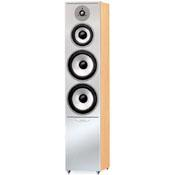QUADRAL Argentum 09 Be the First! WRITE A REVIEW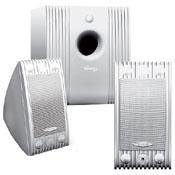QUADRAL MM 220 Be the First! WRITE A REVIEW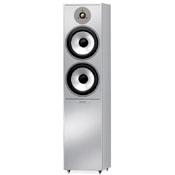QUADRAL Argentum 07 Be the First! WRITE A REVIEW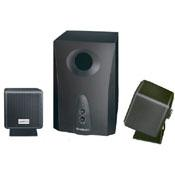QUADRAL SM 2650 Cube Be the First! WRITE A REVIEW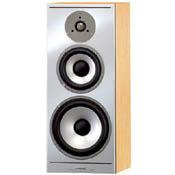QUADRAL Argentum 03 Be the First! WRITE A REVIEW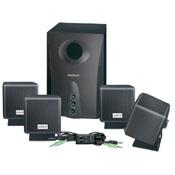QUADRAL SM 4950 Cube Be the First! WRITE A REVIEW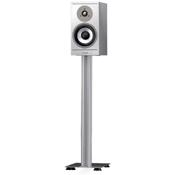QUADRAL Argentum 02 Be the First! WRITE A REVIEW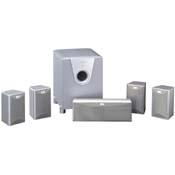QUADRAL Surround 3200 Be the First! WRITE A REVIEW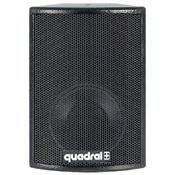QUADRAL PRO 16 Be the First! WRITE A REVIEW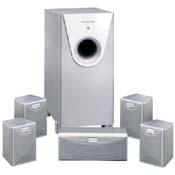QUADRAL Surround 1275 Be the First! WRITE A REVIEW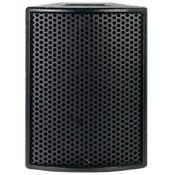QUADRAL PRO 20 Be the First! WRITE A REVIEW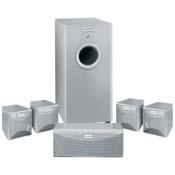QUADRAL Surround 1255 Be the First! WRITE A REVIEW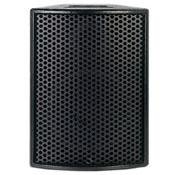QUADRAL PRO 30 Be the First! WRITE A REVIEW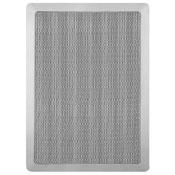QUADRAL MRS 75 Be the First! WRITE A REVIEW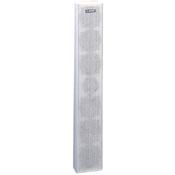QUADRAL SMS 200 WR Be the First! WRITE A REVIEW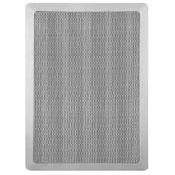QUADRAL MRS 60 Be the First! WRITE A REVIEW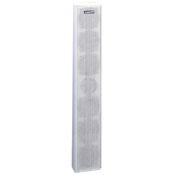QUADRAL SZ 100II Be the First! WRITE A REVIEW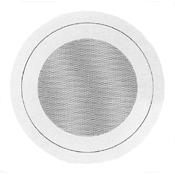QUADRAL XS 6T-WR Be the First! WRITE A REVIEW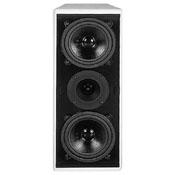QUADRAL SMS 60 Be the First! WRITE A REVIEW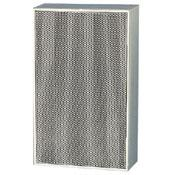QUADRAL 1k-6T WR Be the First! WRITE A REVIEW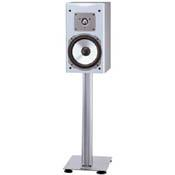QUADRAL Platinum 220 Be the First! WRITE A REVIEW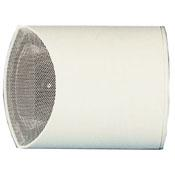QUADRAL CP-6T Be the First! WRITE A REVIEW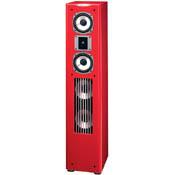QUADRAL Platinum M Be the First! WRITE A REVIEW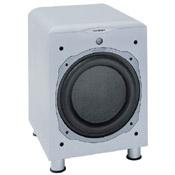QUADRAL SUB 400 DV Be the First! WRITE A REVIEW